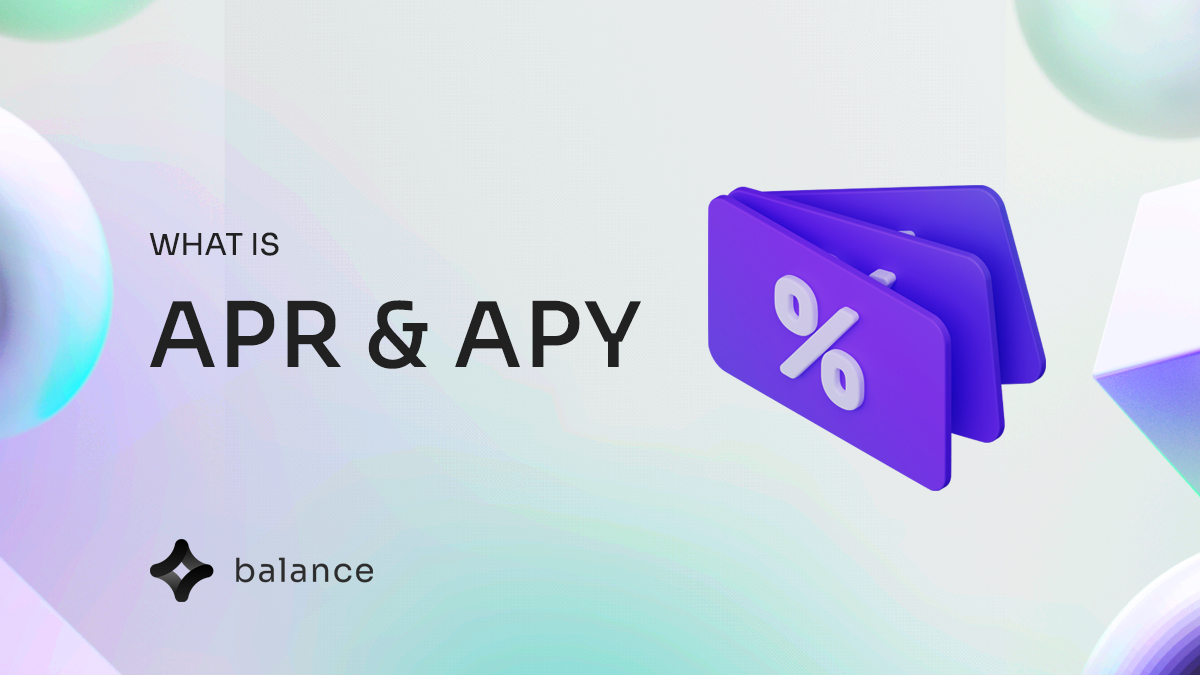October 31, 2022

# APR and APY: Differences and Why They Matter# Balance CapitalIn DeFi, we often hear the terms APR and APY - and yield seekers and passive income investors use these concepts to understand their return on investments over the period of time.

In this article, we will discuss in detail what APR and APY are, how they differ, and then explain why they matter. Let's get started.

# Annual Percentage Rate: APR

Annual Percentage Rate(APR), is the annual rate of return, expressed in percentages and is also known as Simple Interest. APR is what you would earn if you were only paid interest on the principal of your investment and no compound. Therefore if you invest \$1000 and you make 10% APR, at the end of the year you will make \$100.

To calculate APR The following formula can be used:

A = [ P × (1 + R×T) ]

A — total final amount

P — initial investment or loan amount

R — interest rate

T — time in years

# Annual Percentage Yield: APY

APY stands for Annual Percentage Yield, which is a way of expressing the interest rate you earn on your savings, also known as Compound Interest. APY is calculated by taking the interest rate and multiplying it by the number of times that you earn interest during a given time.

For example: If you have \$5,000 worth of FHM that you stake that gives you a 48% APY, you will earn \$2400 an year of passive income and this includes the number of times that the interest made on the \$5000 was compounded. The interest earned in APY can be compounded at any set period for example, daily, weekly, monthly or yearly. Therefore it is a good idea to see what deal you are getting before you stake your tokens.

Below is the formula used to calculate APY:

A = [ P (1 + R/N) N ]

A — total final amount

P — initial investment

R — interest rate

N — number of compounding periods (daily, weekly, monthly, yearly)

So what is the difference between APR and APY?

published

APRYpost

APRs and APYs mainly differ in the fact that APY involves the compounding of the investment, taking into account all other factors such as the initial investment, the stated interest rate, and the investment period are the same.

As a result APY will always result in a higher ROI compared to APR.

There are two things we want you to take away from this article:

• When it comes to taking a loan, prefer using APR rates as the interest you pay back will be lower.
• When it comes to investing/ staking, use APY rates, as your ROI will be higher due to the compounding effect.